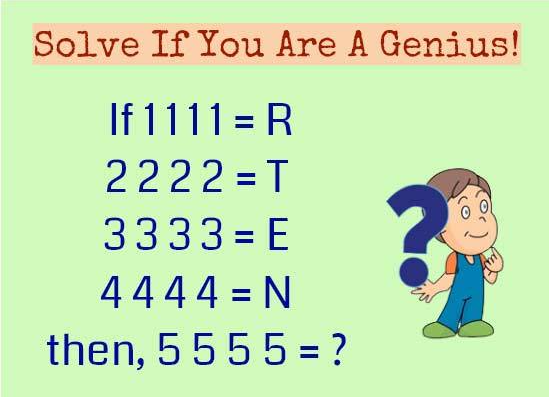# Solve This Math Brainteaser and You Are A Genius

Are you able to solve this puzzle? Let’s see whether you’re one of those wise individuals.If 1 1 1 1 = R

2 2 2 2 = T

3 3 3 3 = E

4 4 4 4 = N

then, 5 5 5 5 = ?

.

.

.

.

.

Here, you need to add the numbers and then write it in words and pick up the last letter.

So, 1 1 1 1 = 4 = Four = The last letter of four is R.

Similarly,2 2 2 2 = 8 = Eight = T

3 3 3 3 = 12 = Twelve = E

4 4 4 4 = 16 = Sixteen = N

Hence,

5 5 5 5 = 20 = Twenty = Y